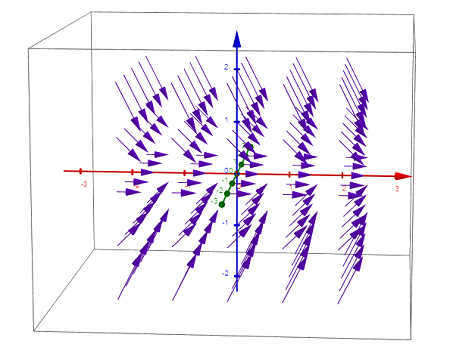# Sketch a vector field for the given function F(x,y,z) = \langle 1, \ 0 , \ z \rangle .

## Question:

Sketch a vector field for the given function

{eq}F(x,y,z) = \langle 1, \ 0 , \ -z \rangle {/eq}.

## Three-dimensional Vector Field:

The vector can be easily sketched with the technology of graphing utility and its capability. The vector field is first given or can be found if its vector function is given. The vector is then plotted in two or three dimensions.

In this question, we have to sketch a vector field for the given function

{eq}F(x,y,z) = \langle 1, \ 0 , \ -z \rangle {/eq}.

So this is sketched using the technology and graphing utility, and is shown below;